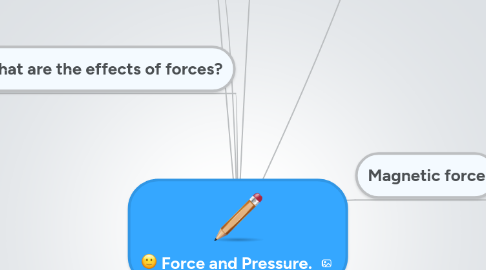# Force and Pressure.

Jetzt loslegen. Gratis!Force and Pressure.## 2. What are the different types of forces ?

### 2.1. The different types of forces are the following :Frictional force,gravitational force and magnetic force.

2.1.1. Magnetic forces and Gravitational forces are non-contact forces .

2.1.2. Frictional force is a contact force

## 3. What are the effects of forces?

### 3.1. change the speed of a moving object either by increasing its speed or slowing down

3.1.1. New node

3.1.1.1. New node

## 5. Frictional force

### 5.5. Friction can also be a problem.Friction is often a nuisance because it makes movement more difficult , and wears away materials .

5.5.1. Another example is , friction between the air and the cyclist slows down the speed of the cyclist.

5.5.2. For example, friction between the tyres and the road has worn out the rubber on these tyres .

### 5.6. Ways to reduce friction:

5.6.1. Having a streamlined body shape such as the ( aeroplanes and the fishes.)

5.6.3. Lubrication

5.6.4. Ball bearings

### 5.7. But still without Friction ,many things in the world would not be possible at all .

5.7.1. For example, friction holds a nail on the wall , Friction between our feet and the ground gives us the grip needed to walk and run,Friction between the wheel and the brakes slows down the bicycle

## 6. Magnetic force

### 6.1. A magnet can attract objects made of iron and steel .The magnet exerts a force called the magnetic force.

6.1.1. Examples of magnetic forces in dailuy life.

6.1.1.1. A magnetic catch for the door of a cabinet keeps the door shut tight.

6.1.1.2. The needle of a compass is a small magnet . A compass is used to give directions

6.1.1.3. A magnet attracts steel pins .Picking up the pins ourselves may easily injure our bare fingers .

6.1.1.4. The crane uses a powerful to lift iron and steel objects.

### 6.2. Forces between magnets

6.2.1. A magnet can also exert a force on the other magnets . Bar magnets have two poles - a north - seeking pole (N) at one end and a south-seeking pole (S) at the other end . When like poles face each other ,the magnets repel (PUSH.) .When unlike poles face each other , the magnets attract (PULL) .

6.2.2. Maglev Train .

6.2.2.1. Magnetic forces are also used in Transportation .

6.2.2.2. 'Maglev' comes from the words 'magnetic levitation' (rising and floating) . Magnets in the track and train repel and lift the train a few centimetres above the track.. As there is no contact between the track and the vehicle , the friction is reduced. This is why ,the 'Maglev Train' is able to move so fast.

## 7. Gravitational force

### 7.1. The weight of an object , depends on the force of gravity pulling on that object.thus weight is the amount of force at gravitational force acting on an object.

7.1.1. When a greater force of gravity is pulling on the object,the object will be heavier. Our mass cannot change whereas our weight can change.For example if a person weighing 60 kg on the Earth, making her weight 600 N ,her weight will only be 100 N.

7.1.1.1. The gravitational pull of the moon is about 1/6 of the Earth's , hence the person's weight differs from place to place.